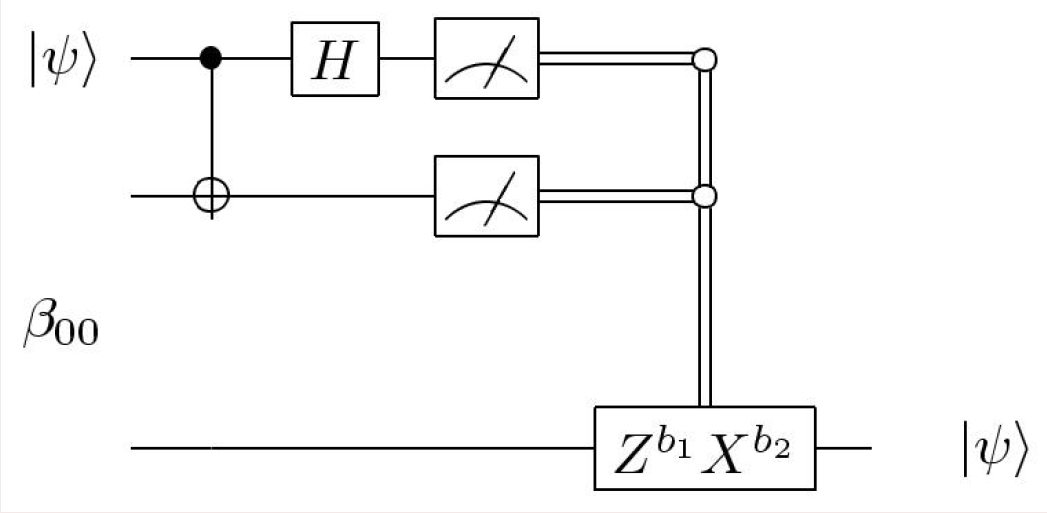# How is the Ebit measurement (or Bell state measurement), if Charlie has an entangled state?

Quantum Computing Asked by 299792458 on December 17, 2020

Assume Charlie has an entangled state like $$psi_{00}|00rangle+psi_{11}|11rangle,$$, and he wants to send one of the share qubits to Alice and then Alice does a Bell state measurement and then tells Bob the result of two bits, and then Bob recovers Charlie’s state. So if Charlie’s state is unentangled and for example one bit like $$|0rangle$$, then it is obvious. But my question is about the situation that Charlie’s state is entangled and then sends one share qubit to Alice. Now, I have some questions according to this circuit:I should also mention that the Bell state of Alice and Bob’s qubits is $$|beta_{00}rangle=(|00rangle+|11rangle)/sqrt2$$.

1. How can I understand the step-by-step change of every single qubit in the below circuit?
2. Assume after recovering Charlie’s state by Bob, he sends it back to Charlie. So now, How can Charlie have his entangled state again (I really do not understand that)?
3. What happens if $$psi_{imath imath} rightarrow 1$$ for each $$imath$$ and $$psi_{00}=psi_{11}$$?

I should mention that I want also the resultant state for the input $$psi_{00}|00rangle+psi_{11}|11rangle,$$ with $$psi_{imath imath}$$ any complex numbers subject to the normalization condition, subject to any one of the four ebit measurements $$left|b_{1} b_{2}rightrangleleftlangle b_{1} b_{2}right|$$, which $$b_1$$ is the share qubit that Alice has and $$b_2$$ is the share qubit that Bob has.

## Related Questions

### Why and how is quantum noise predictable?

1  Asked on February 26, 2021

### Are almost-Clifford circuits almost easy to simulate?

1  Asked on February 26, 2021 by forky40

### How is a quantum simulator able to simulate a quantum mechanical properties on a classical computer?

2  Asked on February 26, 2021 by sinestro-38

### How to fix “QiskitChemistryError:PySCF is not installed”

2  Asked on February 25, 2021 by anneahilator

### How to initialize a random state in Qiskit?

2  Asked on February 24, 2021

### Conditional probability between parameter and operator in quantum mechanics?

0  Asked on February 24, 2021

### Tensor product properties used to obtain Kraus operator decomposition of a channel

1  Asked on February 24, 2021 by alexander-pozdneev

### Connection between smooth max-relative entropy and smooth max-information

0  Asked on February 24, 2021

### Recording the time taken by a VQE to find ground state energy

1  Asked on February 23, 2021 by louisl

### Grover search with different diffusion operators

1  Asked on February 23, 2021

### Has any research been done on quantum Zeno machines?

2  Asked on February 22, 2021 by user820789

### Can I adjust the quantum circuit after transpiling it?

1  Asked on February 22, 2021

### Qiskit sample – Portfolio optimization

1  Asked on February 20, 2021 by pavan-kulkarni

### Expectation value of operator – python

1  Asked on February 18, 2021

### How does one create the unitary sending $|0rangle$ into a target quantum state?

3  Asked on February 18, 2021 by bg827

### How can I convert the unitary matrix $e^frac{ipi}{2}$ into a quantum circuit in Qiskit?

1  Asked on February 18, 2021 by physics-world

### Nielsen & Chuang Exercise 6.13: Standard deviation of classical counting algorithm

1  Asked on February 16, 2021

### Post-selection applied to quantum teleportation

1  Asked on February 16, 2021

### Does normalizing a mixed state give a pure state?

2  Asked on February 16, 2021 by eesh-starryn